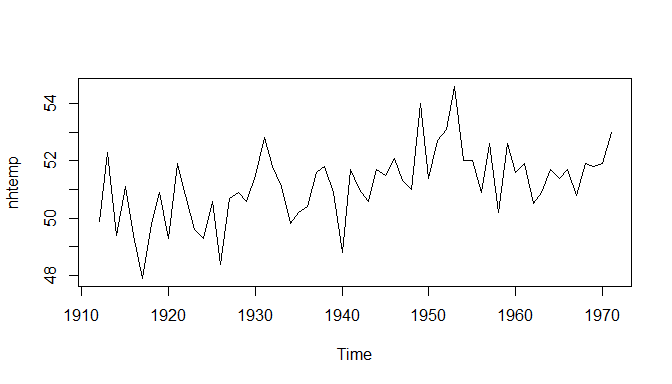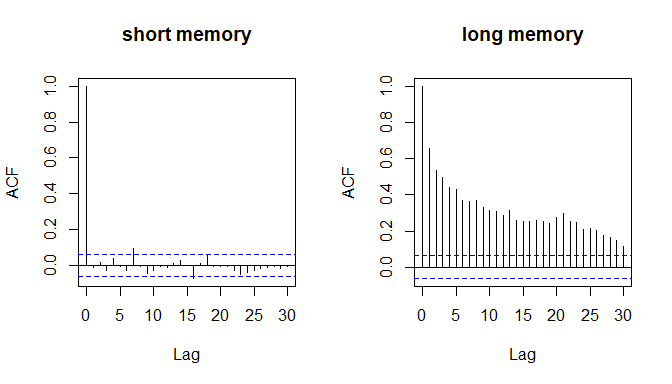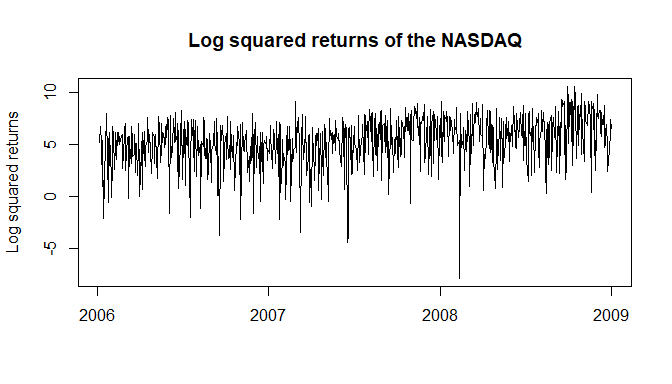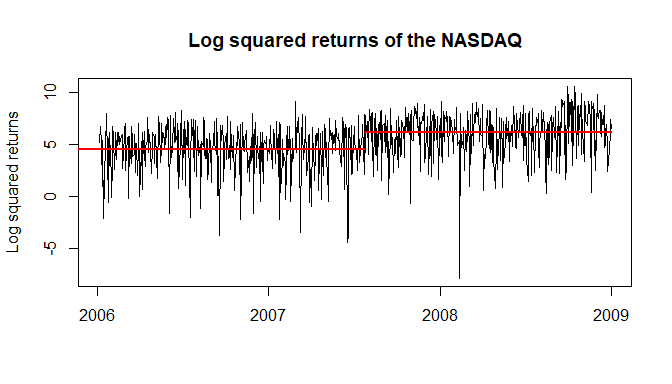# memochange-Tutorial: Change in Mean

#### 2020-07-26

The memochange package can be used for two things: Checking for a break in persistence and checking for a change in mean. This vignette presents the functions related to a change in mean. This includes the functions CUSUMfixed, CUSUMLM, CUSUM_simple, fixbsupw, snsupwald, snwilcoxon, and wilcoxonLM. Before considering the usage of these functions, a brief literature review elaborates on their connection.

## Literature Review

In standard time series models it is usually assumed that the series have a constant mean over time. If this assumption is invalidated, inference and forecasts based on such models are misleading. Therefore, testing for a change in mean is of major importance.

A typical example of a time series that could be subject to a change in mean is the series of average yearly temperatures in New Haven. It is visualized in the following graph.

utils::data(nhtemp)
graphics::plot(nhtemp)Three standard procedures to test for a change in mean at an unknown point in time are CUSUM tests originally proposed by Brown, Durbin, and Evans (1975), Wilcoxon-type rank tests (e.g. Bauer (1972)), and sup-Wald tests by Andrews (1993). Applying the standard CUSUM test, for example, we observe for the above temperature series that the corresponding p-value of the test is smaller than any resonable significance level.

strucchange::sctest(strucchange::efp(nhtemp ~ 1, type = "OLS-CUSUM"))
#>
#>  OLS-based CUSUM test
#>
#> data:  strucchange::efp(nhtemp ~ 1, type = "OLS-CUSUM")
#> S0 = 2.0728, p-value = 0.0003709

Therefore, it rejects the null hypothesis of a constant mean over time and we conclude that there is a change in mean.

However, all these standard tests suffer from the issue that they cannot be applied under long memory. In a persistent long-memory time series far distant observations are significantly correlated. The degree of persistence is given by the long-memory parameter $$d \in [0,0.5)$$. Higher values of $$d$$ indicate higher persistence of the series. The special case $$d=0$$ is called short memory where far distant observations are not significantly correlated anymore. The difference between a short-memory and a long-memory time series can be seen easily in the autocorrelation function (acf) of a series, which gives the correlation between observations separated by various time lags.

T            <- 1000
series_short <- fracdiff::fracdiff.sim(n=T,d=0)$series series_long <- fracdiff::fracdiff.sim(n=T,d=0.45)$series

graphics::par(mfrow=c(1,2))
stats::acf(series_short,main="short memory")
stats::acf(series_long,main="long memory")We observe that the acf of the short-memory time series dies out quickly, while the autocorrelations in the long-memory time series are even at a very large lag (i.e. for far distant observations) high.

The above mentioned standard tests for a change in mean are developed under short memory. Wright (1998) and Krämer and Sibbertsen (2002), among others, found that they asymptotically reject the null hypothesis of a constant mean with probability one under long memory. This can be seen in a simple Monte Carlo simulation for the standard CUSUM test. We simulate $$N$$ times a short-memory ($$d=0$$) and a long-memory ($$d=0.45$$) time series of length $$T$$ without any change in mean, apply the test, and investigate how often the null hypothesis is rejected with a nominal significance level of $$5\%$$. Therefore, since we are under the null hypothesis we expect on average $$5\%$$ rejections.

set.seed(410)
T                  <- 500
N                  <- 500
results_short      <- vector("numeric",N)
results_long       <- vector("numeric",N)
for(i in 1:N)
{
series_short     <- fracdiff::fracdiff.sim(n=T,d=0)$series series_long <- fracdiff::fracdiff.sim(n=T,d=0.45)$series
results_short[i] <- strucchange::sctest(strucchange::efp(series_short ~ 1, type = "OLS-CUSUM"))$p.value<0.05 results_long[i] <- strucchange::sctest(strucchange::efp(series_long ~ 1, type = "OLS-CUSUM"))$p.value<0.05
}
mean(results_short)
#>  0.056
mean(results_long)
#>  0.992

Under short memory the test roghly holds its significance level of $$5\%$$. However, under long memory the standard CUSUM test nearly always rejects. It cannot be used whenever $$d>0$$.

Due to the problems described, a lot of researchers modified standard testing procedures for a change in mean to account for $$0<d<0.5$$ in recent years. A review is given in Wenger, Leschinski, and Sibbertsen (2019). Tests based on the CUSUM testing principle are the CUSUM-LM test by Horváth and Kokoszka (1997) and Wang (2008), the (simple) CUSUM test based on fractionally differenced data by Wenger, Leschinski, and Sibbertsen (2018), and the CUSUM fixed bandwidth tests by Wenger and Leschinski (2019). Wilcoxon type tests are the Wilcoxon-LM test by Dehling, Rooch, and Taqqu (2013) and the self-normalized Wilcoxon test by Betken (2016). Modified sup-wald tests for a change in mean are the self-normalized sup-Wald test by Shao (2011) and the fixed-b sup-Wald test by Iacone, Leybourne, and Taylor (2014).

The tests can be further divided into more or less three groups depending on how the variance of the mean (long-run variance) that appears in the denominator of all test statistics is estimated. Without going into too many details, the first group (CUSUM-LM and Wilcoxon-LM tests) utilizes the MAC estimator by Robinson (2005) that consistently estimates the long-run variance under long memory. The second group (self-normalized Wilcoxon and self-normalized sup-Wald tests) applies a self-normalization approach (see Shao (2010)). A self-normalizer is not a consistent estimate of the long-run variance, but proportional to it, even when $$d>0$$. The third group (CUSUM fixed bandwidth and fixed-b sup-Wald tests) uses fixed bandwidth approach (see Kiefer and Vogelsang (2005) and Hualde and Iacone (2017)). It is a generalization of the self-normalization approach.

Wenger, Leschinski, and Sibbertsen (2019) observe via simulations that for fractionally integrated White noise time series (which is one class of long-memory time series) the CUSUM testing procedure seems to offer the highest rejection rates under the alternative of a mean shift (i.e. offers the highest power). However, the first group of tests that apply a consistent estimator of the long-run variance (e.g. the CUSUM-LM test) also rejects the null hypothesis of a constant mean too often in a time series that is not subject to a mean shift. In other words these tests are often size distorted. In contrast, the self-normalized and fixed bandwidth tests hold their size in most of the situations. For fractionally integrated heavy tailed time series (which is another class of long-memory time series) it is shown by Dehling, Rooch, and Taqqu (2013) and Betken (2016) that Wilcoxon type tests are superior to CUSUM tests.

The simple CUSUM test by Wenger, Leschinski, and Sibbertsen (2018) is a little bit exceptional in the list of tests implemented. The reason is that instead of modifying the standard CUSUM test it modifies the data the standard test is applied on.

## Usage

Two examples how to conduct the change-in-mean tests implemented in the memochange package are discussed in the following. The first example is an application of the tests to a real data set. The second example is a small Monte Carlo simulation where the performance of the tests is compared.

First, we consider the log squared returns of the NASDAQ in the time around the global financial crisis (2006-2009). We download the daily stock price series from the FRED data base.

nasdaq=data.table::fread("https://fred.stlouisfed.org/graph/fredgraph.csv?bgcolor=%23e1e9f0&chart_type=line&drp=0&fo=open%20sans&graph_bgcolor=%23ffffff&height=450&mode=fred&recession_bars=on&txtcolor=%23444444&ts=12&tts=12&width=1168&nt=0&thu=0&trc=0&show_legend=yes&show_axis_titles=yes&show_tooltip=yes&id=NASDAQCOM&scale=left&cosd=2006-01-01&coed=2009-01-01&line_color=%234572a7&link_values=false&line_style=solid&mark_type=none&mw=3&lw=2&ost=-99999&oet=99999&mma=0&fml=a&fq=Daily&fam=avg&fgst=lin&fgsnd=2009-06-01&line_index=1&transformation=lin&vintage_date=2019-11-04&revision_date=2019-11-04&nd=1971-02-05")

Next calculate the log squared returns as a measure of volatility and plot the series.

nasdaq[NASDAQCOM==".", NASDAQCOM := NA]
nasdaq              <- as.data.frame(nasdaq)
nasdaq              <- stats::na.omit(nasdaq)
nasdaq$NASDAQCOM <- as.numeric(nasdaq$NASDAQCOM)
nasdaq$DATE=zoo::as.Date(nasdaq$DATE)
nasdaq_xts=xts::xts(nasdaq[,-1],order.by = nasdaq$DATE) #> Registered S3 method overwritten by 'xts': #> method from #> as.zoo.xts zoo nasdaq_xts <- log(diff(nasdaq_xts)^2)[-1] graphics::par(mfrow=c(1,1)) zoo::plot.zoo(nasdaq_xts, xlab="", ylab="Log squared returns", main="Log squared returns of the NASDAQ")A first visual impression is that the mean seems to increase in the second part of the sample. Furthermore, applying the local Whittle estimator (choosing the bandwidth as T^0.65, which is usual in literature) we observe that there is the potential that the time series possess high persistence (d>0). T <- length(nasdaq_xts) x <- as.numeric(nasdaq_xts) d_est <- LongMemoryTS::local.W(x, m=floor(1+T^0.65))$d
round(d_est,3)
#>  0.303

Therefore, as discussed above the standard testing procedures for a change in mean cannot be applied. Instead, one of the functions CUSUM_simple, CUSUMfixed, CUSUMLM, fixbsupw, snsupwald, snwilcoxon, and wilcoxonLM have to be used. The functionality of all tests is similar. They require a univariate numeric vector x as an input variable and yield a matrix of test statistic and critical values as an output variable.

We apply the CUSUM fixed-m type A test of Wenger and Leschinski (2019) implemented in the function CUSUMfixed as an example. First, the arguments of the function are explained since it nests all arguments of the other implemented functions to test for a change in mean in a persistent time series.

We have to insert the (estimated) long-memory parameter d as a first argument. The critical values of all tests depend on the long-memory parameter, except for the simple CUSUM test. However, also in the function CUSUM_simple the long-memory parameter has to be inserted since it is used to transform the time series the test is applied on.

As a second argument the type of the CUSUM fixed bandwidth function has to be supplied. The user can choose between the CUSUM fixed-b and fixed-m tests of type-A or -B. According to Wenger and Leschinski (2019) the type-A tests outperform the type-B tests when the break is in the middle of the series while the revearse is true when the break break occurs at the beginning or the end of the series.

In all fixed bandwidth functions (CUSUMfixed, fixbsupw) the bandwidth bandw has to be chosen. The bandwidth determines how many autocovariances for the fixed-$$b$$ tests and respectively how many periodogram ordinates for the fixed-$$M$$ tests are included in the estimators of the long-run variance. For the fixed-$$b$$ tests $$b\in(0,1]$$ and for the fixed-$$M$$ tests $$M\in[1,T]$$. Since the critical values of all fixed bandwidth tests depend not only on $$d$$, but also on the bandwidth, just for a couple of bandwidths critical values are given in the functions. Wenger and Leschinski (2019) and Iacone, Leybourne, and Taylor (2014) suggest to use $$b=0.1$$ and $$M=10$$.

The last argument of all tests is tau. It corresponds to the search area $$[\tau,1-\tau]$$ with $$\tau \in (0,1)$$, in which the test statistics are calculated. Andrews (1993) suggests using $$\tau_{1}=1-\tau_{2}=0.15$$, which is the default value for tau. Note that critical values of the tests are also dependent on tau and just implemented for the default value.

Executing the test we get the following result.

library(memochange)
CUSUMfixed(x,d=d_est,procedure="CUSUMfixedm_typeA",bandw=10)
#>           90%           95%           99% Teststatistic
#>         1.499         1.615         1.805         1.931

The output of all functions is a matrix consisting of the test statistic and critical values for the null hypothesis of a constant mean against the alternative of a change in mean at some unknown point in time. Here, the results suggest that a change in mean has occurred somewhere in the series since the test statistic exceeds the critical value at the one percent level.

To correctly model and forecast the series, the exact location of the break is important. This can be estimated by the breakpoints function from the strucchange package.

BP       <- strucchange::breakpoints(x~1)$breakpoints BP_index <- zoo::index(nasdaq_xts[BP]) BP_index #>  "2007-07-23" The function indicates that there is a break in persistence in July, 2007, which roughly corresponds to the start of the world financial crisis. The following plot shows the time series and the estimated means before and after the break T_index <- zoo::index(nasdaq_xts[T]) m1 <- mean(nasdaq_xts[1:BP]) m2 <- mean(nasdaq_xts[(BP+1):T]) zoo::plot.zoo(nasdaq_xts, xlab="", ylab="Log squared returns", main="Log squared returns of the NASDAQ") graphics::segments(0,m1,BP_index,m1,col=2,lwd=2) graphics::segments((BP_index+1),m2,T_index,m2,col=2,lwd=2)As a second example, we compare the performance of two of the implemented tests via a Monte Carlo simulation study. Under the null hypothesis (i.e. if there is no shift in the series) the tests should reject in $$\alpha \%$$ of cases. Here, $$\alpha$$ is the significance level and we choose $$\alpha=0.05$$. Under the alternative (i.e. if there is a shift in the series), the tests should reject in most of the cases (at best: always). When the length of the time series increases, the rejection rates should increase. We simulate fractionally integrated White noise time series (with and without breaks) using the fracdiff.sim function from the fracdiff package. To estimate the memory parameter we apply the local Whittle estimator by Robinson (1995) using the local.W function from the LongMemoryTS package. The setup is very similar to the published paper by Wenger and Leschinski (2019). The simulation can be extended by all other change-in-mean tests that are implemented in the memochange package, which is not been done here to save computing time.  test_func<-function(T,d) { # Simulate a fractionally integrated (long-memory) time series of # length T with memory d that is not subject to a shift. tseries <- fracdiff::fracdiff.sim(n=T,d=d)$series

# Simulate a fractionally integrated (long-memory) time series of
# length T with memory d that is subject to a shift in the middle of
# the sample of magnitude 2.
changep     <- c(rep(0,T/2),rep(2,T/2))
tseries2    <- tseries+changep

# Estimate the long-memory parameter of both series using the suggested bandwidth.
d_est       <- LongMemoryTS::local.W(tseries, m=floor(1+T^0.65))$d d_est2 <- LongMemoryTS::local.W(tseries2, m=floor(1+T^0.65))$d

# Apply both functions on both time series. Arguments are chosen according to
# Wenger, Leschinski (2019) who propose these tests.
typeAsize   <- CUSUMfixed(tseries,d=d_est,procedure="CUSUMfixedm_typeA",bandw=10)
typeBsize   <- CUSUMfixed(tseries,d=d_est,procedure="CUSUMfixedm_typeB",bandw=10)
typeApower  <- CUSUMfixed(tseries2,d=d_est2,procedure="CUSUMfixedm_typeA",bandw=10)
typeBpower  <- CUSUMfixed(tseries2,d=d_est2,procedure="CUSUMfixedm_typeB",bandw=10)

# Save if the tests reject at the 5% significance level.
decAsize    <- typeAsize["Teststatistic"] > typeAsize["95%"]
decBsize    <- typeBsize["Teststatistic"] > typeBsize["95%"]
decApower   <- typeApower["Teststatistic"] > typeApower["95%"]
decBpower   <- typeBpower["Teststatistic"] > typeBpower["95%"]

return(c(decAsize,decBsize,decApower,decBpower))
}

In the next step the Monte Carlo simulation ($$N=500$$ replications) is executed. The parameters we use for the simulated fractionally integrated White noise time series are series length $$T=[50,100]$$ and long-memory parameters $$d=[0.1,0.2]$$.

set.seed(410)
# Parameter setting considered
T_grid              <- c(50,100)
d_grid              <- c(0.1,0.2)
N                   <- 500

# Generate array to save the results
resultmat           <- array(NA, dim=c(length(T_grid),length(d_grid),4))
dimnames(resultmat) <- list(paste("T=",T_grid,sep=""),paste("d=",d_grid,sep=""),
paste(rep(c("type-A","type-B"),2),c("size","size","power","power"),sep=" "))

# Monte Carlo simulation
for(TTT in 1:length(T_grid))
{
T <- T_grid[TTT]
for(ddd in 1:length(d_grid))
{
d                 <- d_grid[ddd]
result_vec        <- 0
for(i in 1:N)
{
result_vec        <- result_vec+test_func(T,d)
}
resultmat[TTT,ddd,] <- result_vec/N
}
}
# Results
resultmat
#> , , type-A size
#>
#>       d=0.1 d=0.2
#> T=50  0.020 0.032
#> T=100 0.026 0.046
#>
#> , , type-B size
#>
#>       d=0.1 d=0.2
#> T=50  0.016 0.028
#> T=100 0.036 0.046
#>
#> , , type-A power
#>
#>       d=0.1 d=0.2
#> T=50   0.86 0.824
#> T=100  1.00 0.960
#>
#> , , type-B power
#>
#>       d=0.1 d=0.2
#> T=50  0.830 0.770
#> T=100 0.998 0.938

We observe that both tests do not exceed $$\alpha=5\%$$ rejections when no break occured in the long-memory time series (first two tables). Furthermore, the type-A test rejects more often than the type-B test the null hypothesis when a shift occured. Therefore, this small Monte Carlo simulation leads to the same conclusion as the paper of Wenger and Leschinski (2019), i.e. that the type-A test outperforms the type-B test for fractionally integrated White noise time series when the break is in the middle of the series.